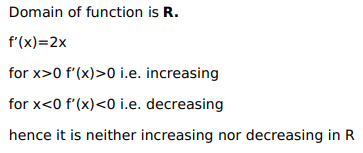# Solve this following

Question:

Show that the function $f(x)=\mathrm{X}^{2}$ is

a. strictly increasing on $[0, \infty[$

b. strictly decreasing on $[0, \infty[$

c. neither strictly increasing nor strictly decreasing on $R$

Solution: